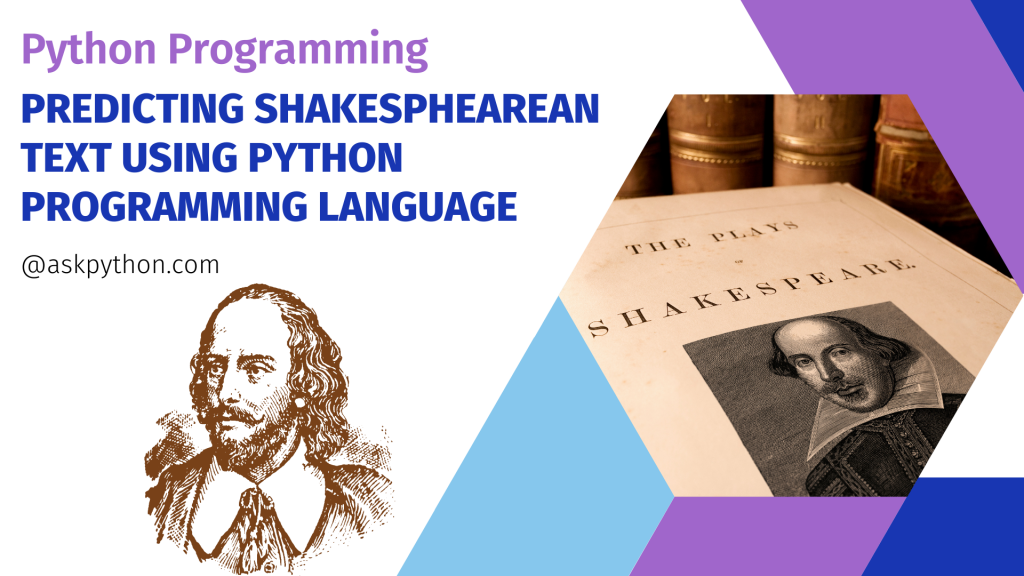# Predict Shakespearean Text Using Keras TensorFlowHey folks! In this tutorial, we will look at how to use the Keras TensorFlow API in Python to create a Recurrent Neural Network model to predict Shakespearean text.

Also read: Stock Price Prediction using Python

To produce fresh text, we will train the GitHub Shakespearean text dataset using a custom-built RNN model.

## Step 1: Importing Libraries

We utilized some of the most popular deep learning libraries. Sweetviz is a new package that automates exploratory data analysis and is particularly beneficial in analyzing our training dataset.

```pip install sweetviz
import numpy as np
import pandas as pd
import matplotlib.pyplot as plt
import tensorflow as tf
from tensorflow import keras
import sweetviz as sw
import seaborn as sns
sns.set()
```

```shakespeare_url='https://raw.githubusercontent.com/karpathy/char-rnn/master/data/tinyshakespeare/input.txt'
filepath=keras.utils.get_file('shakespeare.txt',shakespeare_url)
with open(filepath) as f:
```
```Downloading data from https://raw.githubusercontent.com/karpathy/char-rnn/master/data/tinyshakespeare/input.txt
1122304/1115394 [==============================] - 0s 0us/step
1130496/1115394 [==============================] - 0s 0us/step
```

Now that we’ve downloaded the dataset into our Python notebook, we need to preprocess it before we can utilize it for training.

## Step 3: Pre-processing the Dataset

Tokenisation is the process of dividing lengthy text strings into smaller portions or tokens. Larger chunks of text can be tokenized into sentences, and then into words.

Pre-processing will also involve removing punctuations from the tokens generates as well.

```tokenizer=keras.preprocessing.text.Tokenizer(char_level=True)
tokenizer.fit_on_texts(shakespeare_text)

max_id=len(tokenizer.word_index)
dataset_size=tokenizer.document_count
[encoded]=np.array(tokenizer.texts_to_sequences([shakespeare_text]))-1
```

## Step 4: Preparing the Dataset

We will be using `tf.data.Dataset` which is generally useful for a large set of elements like huge chunks of textual data.

`Dataset.repeat()` goes over the dataset and repeats the dataset a specified number of times. `window()` is like a sliding window that slides the window by a specified number each time for repeated iteration.

```train_size=dataset_size*90//100
dataset=tf.data.Dataset.from_tensor_slices(encoded[:train_size])

n_steps=100
window_length=n_steps+1
dataset=dataset.repeat().window(window_length,shift=1,drop_remainder=True)

dataset=dataset.flat_map(lambda window: window.batch(window_length))

batch_size=32
dataset=dataset.shuffle(10000).batch(batch_size)
dataset=dataset.map(lambda windows: (windows[:,:-1],windows[:,1:]))
dataset=dataset.map(lambda X_batch,Y_batch: (tf.one_hot(X_batch,depth=max_id),Y_batch))
dataset=dataset.prefetch(1)
```

## Step 5: Building the Model

The model building is pretty simple. We will be creating a sequential model and adding layers to the model with certain characteristics.

```model=keras.models.Sequential()
```

Next, we will be compiling the model and fitting the model on the dataset. We will be using `Adam` optimizer but you can also use other available optimizers according to your preferences.

```model.compile(loss='sparse_categorical_crossentropy',optimizer='adam')
history=model.fit(dataset,steps_per_epoch=train_size // batch_size,epochs=1)
```
```31370/31370 [==============================] - 1598s 51ms/step - loss: 0.9528
```

## Step 6: Testing the Model

We have defined some functions in the code snippet mentioned below. The functions will preprocess and prepare the input data according to our defined model and predict the next characters up to the specified number of characters.

```def preprocess(texts):
X=np.array(tokenizer.texts_to_sequences(texts))-1
return tf.one_hot(X,max_id)

def next_char(text,temperature=1):
X_new=preprocess([text])
y_proba=model.predict(X_new)[0,-1:,:]
rescaled_logits=tf.math.log(y_proba)/temperature
char_id=tf.random.categorical(rescaled_logits,num_samples=1)+1

def complete_text(text,n_chars=50,temperature=1):
for _ in range(n_chars):
text+=next_char(text,temperature)
return text
```

Let’s predict the text for a certain letter or a word using the code mentioned below.

```print("Some predicted texts for letter 'D' are as follows:\n ")
for i in range(3):
print(complete_text('d'))
print()
```
```Some predicted texts for letter 'D' are as follows:

d, swalld tell you in mine,
the remeiviss if i shou

dima's for me, sir, to comes what this roguty.

dening to girl, ne'er i was deckong?
which never be
```
```print("Some predicted texts for word 'SHINE' are as follows:\n ")
for i in range(3):
print(complete_text('shine'))
print()
```

Output:

```Some predicted texts for word 'SHINE' are as follows:

shine on here is your viririno penaite the cursue,
i'll

shine yet it the become done to-k
make you his ocrowing

shine dises'-leck a word or my head
not oning,
so long
```

## Conclusion

Congratulations! You just learned how to build a Shakespearean text predictor using RNN. Hope you enjoyed it! 😇

Liked the tutorial? In any case, I would recommend you to have a look at the tutorials mentioned below:

Thank you for taking your time out! Hope you learned something new!! 😄